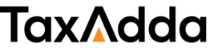# What is Depreciation? Meaning, Methods, Calculations

## What is Depreciation?

Depreciation is the reduction in the value of assets due to wear and tear.

Every asset is subject to wear and tear in the ordinary course of its use and also with the passage of time. The cost of the asset is allocated over time and considered as expense.

It is applied on long term assets which give benefits for many years. For example on plant & machinery, vehicles, computers, furniture, building etc. Land is not subject to wear and tear and thus depreciation is not levied on land but applicable on a building.

Considering depreciation as an expense is very much required for successful financial management. For example, a driver gives his car for tourism purpose, he has to consider the fact that car has a limited useful life and he needs to replace that after some years. For that, while calculating its operation cost, he has to consider the cost of car, its life, the resale value after useful life and add it to other expenses for calculating total cost.

Depreciation is also allowed as an expense as per income tax act and also as per companies act. Although method of calculation is different under both acts and this difference also leads to creation of Deferred tax asset or deferred tax liability.

## Common Methods or Types of Depreciation

Written Down Value (WDV) Method

WDV method  is the most common used method of depreciation. Also in income tax act, depreciation is allowed as per WDV method only.

In this method depreciation is charged on the book value of asset and book value is decreased each year by the depreciation.For eg- Asset is purchased at rs. 1,00,000 and depreciation rate is 10% then first year depreciation is rs. 10,000(10% of rs. 1,00,000), second year depreciation is rs. 9,000 ( 10% of 90,000 [1,00,000 – 10,000]) and third year depreciation is rs. 8,100 ( 10% of rs. 81,000 [90,000 – 9,000]).

This method is also called reducing balance method. In the WDV method, the amount of depreciation goes on decreasing with time. An asset gives more value to a business in initial years then later year, therefore, this method is considered as the most logical method of depreciation.

Formula for calculating depreciation rate (WDV) = {1 – (s/c)^1/n } x 100
n = Remaining useful life of the asset (in years)
s = Scrap value at the end of useful life of the asset
c= Cost of the asset/Written down value of the asset

Straight Line Method (SLM)

In this method, equal amount of depreciation is charged on the asset over its useful life. For Example – asset is purchased for rs. 1,00,000 and useful life is 10 years with salvage value of Rs. 10,000 then depreciation is charged at Rs. 9,000 for each of the 10 years. (1,00,000 – 10,000)/10.

Formula for calculating depreciation rate (SLM) = (100 – % of resale value of purchase price)/Useful life in years
Depreciation = Purchase Price * Depreciation Rate or (Purchase price – Salvage Value)/Useful Life

There are also other methods of depreciation but they are not often used such as depreciation on the basis of units of production.

In companies act the depreciation rate is also based on the number of shifts. Logically an asset is expected to have a shorter life if it used extensively.

Example –

Cost of asset = 2,00,000
Salvage value = 30,000
Useful Life = 10 Years

And thus Depreciation rate as per SLM = (100-15)/10 = 8.5%
Depreciation rate as per WDV = 17.28

 Year Depreciation as per SLM Depreciation as per WDV 1 17,000 34,560.53 2 17,000 28,588.38 3 17,000 23,648.23 4 17,000 19,561.75 5 17,000 16,181.43 6 17,000 13,385.24 7 17,000 11,072.23 8 17,000 9,158.92 9 17,000 7,576.24 10 17,000 6,267.04 Total Depreciation 1,70,000 1,70,000

## Accounting for Depreciation

Depreciation is an expense and reduces the book value of an asset. Therefore a simple journal entry is to be passed at the end of the year. For example

Depreciation A/c Dr    10,000
To Computer A/c             10,000

Thus depreciation is shown as an Indirect expense in the debit side of profit and loss account and asset’s value is to be shown after the reduction of depreciation in the balance sheet.

There is also another method of accounting for depreciation, although it is rarely used. In this method rather than reducing the value of asset another account is credited named as Accumulated depreciation and depreciation for all assets are transferred into it. It is then shown as a negative item in Fixed asset is balance sheet.

## Benefits/Need of charging depreciation

1. Tax Benefit – Depreciation is allowed as an expense under Income tax and therefore it is important to consider it to save income tax.
2. Mandatory under companies act – It is compulsory to charge depreciation in profit and loss account in companies act 2013.
3. Real Profit – If it is not considered then expenditure on behalf of fixed assets is not considered and the profit may be shown as a high number especially in industries required large plant and machinery. Also, this may lead to high distribution of earnings to shareholders and thus non-availability of funds when business is in need to replace the asset.#### Prateek Agarwal

I am Practicing Chartered Accountant from Jaipur and in practice for more than 9 years. I writes mainly for GST and Finance.

## Are you a CA or GST Practioner?Manage your GST Practice in an easy way

Leave all hassles of Excel/Google Sheets